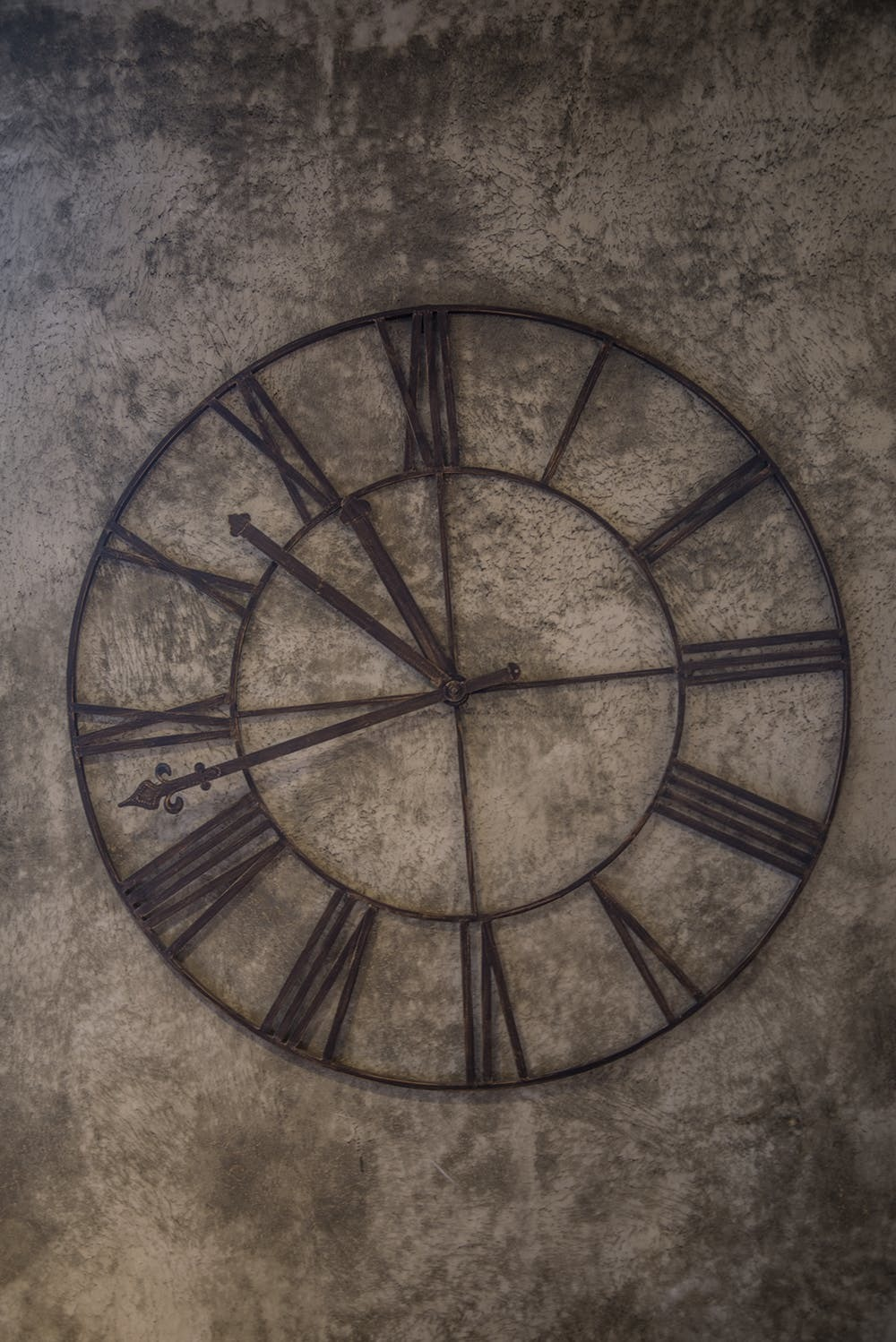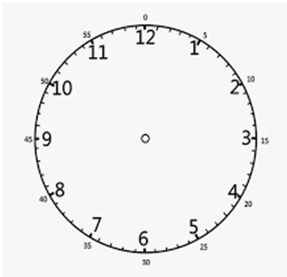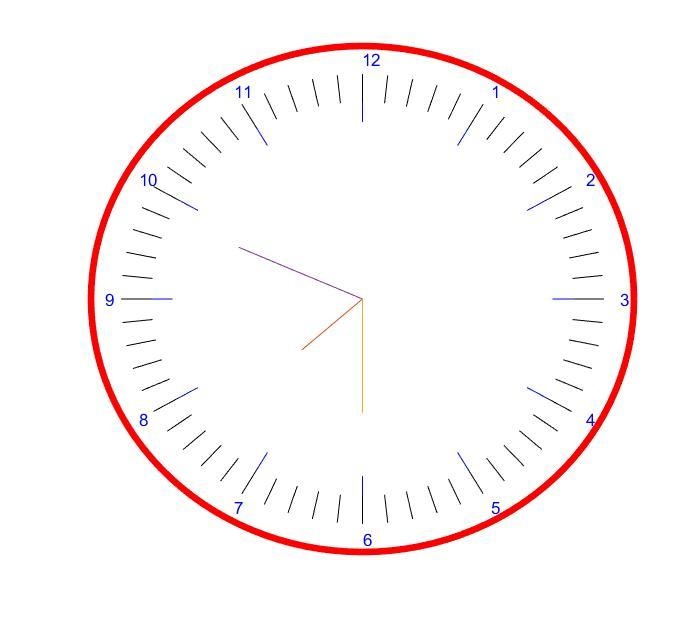# Designing Analog Clock Using MATLAB

May 21, 2020

By  Abhishek Agrawal

`lang: en_US`

NASA might get a fancy digital-count display and retire the analog clock from the Apollo era, but the passion for the analog clock does not end. An analog clock has moving hands, where the smaller one is the hour's hand and travels 30° in one hour, and the longer one is the minute's hand and travels 360° in an hour. The second's hand rotates with a step of 6°. Here we look to design an analog clock using MATLAB.

If you have an analog watch, it tells the time with hands that sweep around a dial: the position of the hands is a measurement of the time. How much the hands move is directly related to what time it is. So if the hour hand sweeps across two segments of the dial, it's showing that twice as much time has elapsed compared to if it had moved only one segment. The point is that the hand's movements over the dial are a way of representing passing time. It's not the same thing as time itself: it's a representation or an analogy of time.

Just because digital technology has advantages, that doesn't mean it's always better than analog. An analog watch might be far more accurate than a digital one if it uses a high-precision movement (gears and springs) to measure time passing. If it has a sweeping second hand, it represents the time more precisely than a digital watch whose display shows only hours and minutes. Surprisingly, analog watches can also keep time better than quartz ones.

Generally, the most expensive watches in the world are analog ones (of course, that's partly because people prefer the way they look), though the world's most accurate atomic clocks show time with digital displays.Fig-1: Analog Clock

The code developed using MATLAB version R2019a. MATLAB has a good collection of graphics commands for plotting and analyzing complex signals. The built-in functions reduce the size of the program and provide the desired output.

### MATLAB Code:

% Author: Abhishek Agrawal
% Topic : Designing Analog Clock using MATLAB
% Company: MATLAB Helper
% Website: https://matlabhelper.com
% Date : 12-05-2020
% With the help of this script we will design an analog clock
% NOTE: CLOCK STARTS AND IS MAINTAINED ACCORDING TO TIME INDICATED BY THE INBUILT COMMAND "clock"
% IMPORTANT NOTE: USE s IN COMMAND LINE TO END THE PROGRAM or Stop the clock
%%%%%%%%%%%%%%%%%%%%%%%%%%%%%%%%%%%%%
%% Clearing the command window and workspace and close all other tabs
clc
close
clear
warning off
%% To create a display of the clock (outer circle)
format shortg
% Creating a circle of radius 10 with centre (0,0)
x=0;y=0;r=10;
hold on;
% angle assigning theta = 0:2*pi i.e. creating a vector theta from 0 to 2*pi
theta = 0:pi/60:2*pi;
% visualization creating and plotting a circle or display for the clock
xc = r * cos(theta);
yc = r * sin(theta);
h = plot(xc,yc,'r','linewidth',4);
axis off
%% To create an analog display for the clock
r=9; i=1;
set(gca,'FontWeight','bold');
% plotting the no. 1 to 12 in the clock every 30 degree apart
for theta = pi/6: pi/6: 2*pi
y1 = r * cos(theta);
x1 = r * sin(theta);
% plotting the lines for 1 to 12
plot([x1/9*8 x1/9*7],[y1/9*8 y1/9*7],'color',[0 0 1])
%num2str converts no. to string to write numbers 1 to 12 in the plot
text(x1/9*9.5,y1/9*9.5,num2str(i),'color',[0 0 1]);
i=i+1;
end
for theta=pi/30 : pi/30 : 2*pi
y1 = 10 * cos(theta);
x1 = 10 * sin(theta);
% plotting the lines for 1 to 12
plot([x1/9*8 x1/9*7],[y1/9*8 y1/9*7],'color',[0 0 0])
end
%% Getting the time from the system
while(1)
tic
% clock returns the 6 element vector containing date and time
c = clock;
% 4:6 for hour, min,sec
c = c(1,4:6);
minute =c(1,2); sec=c(1,3);
% 24hrs time format, if hr >12 so hr-12
if (c(1,1)>12)
hr = c(1,1)-12;
else
hr = c(1,1);
end
min1 = ceil(minute/12);
% Angle of the hour hand
theta = (hr*pi)/6 + (min1*pi)/30;
f=figure(1); hold on;
% Ploting the hour hand
y1 = 3 * cos(theta); Yhr = [0 y1];
x1 = 3 * sin(theta); Xhr = [0 x1];
hrhnd=plot(Xhr,Yhr);hold on;
% Angle sustained by the minute hand
theta1 = (minute*pi)/30;
% Ploting the minute hand
y2 = 4.5 * cos(theta1); Ymin = [0 y2];
x2 = 4.5 * sin(theta1); Xmin = [0 x2];
minhnd=plot(Xmin,Ymin);
% Angle sustained by the second hand
theta2 = (sec*pi)/30;
% Ploting the second hand
y3 = 5 * cos(theta2); Ysec = [0 y3];
x3 = 5 * sin(theta2); Xsec = [0 x3];
sechnd=plot(Xsec,Ysec);
% tic and toc is used in order to get the processing time of loop
z=toc;
% Viewing or pausing the screen for 'x' second
pause(1-z);
% delete all the current figures.
delete(sechnd);
delete(minhnd);
delete(hrhnd);
s = figure(1);
isKeyPressed = ~isempty(get(s,'CurrentCharacter'));
if isKeyPressed
close figure 1;
break;
end
end

A circle with a radius of 10 units is drawn or plotted. Hours are marked from 1 to 12, 30° apart. First, the numbers are converted to string format by using an inbuilt function in MATLAB, i.e.num2strand then by using inbuilt ‘text’ function in MATLAB 1 to 12 is written as text in the plot. System time such as your PC’s is read by the command ‘clock’ which is an inbuilt function in MATLAB. According to the current time of your PC, the hours, minutes, and seconds hands are displayed. As the clock function returns time in 24-hour format, the first step is to convert time to 12-hour format.Fig-2: Display for Analog clock

The minute hand moves 360 degrees or 2 π radians in 60 minutes (or 6 degrees in one minute) and hour hand moves 360 degrees in 12 hours (or 0.5 degrees in 1 minute).

In h hours and m minutes, the minute hand and hour hand would move by :

Minute_hand= (h*60 + m)*6 degrees

Hour_hand=  (h*60 + m)*0.5 degrees

The second-hand moves 360 degrees or 2 π radians in 60 seconds (6 degrees in one second), in ‘s’ seconds, the second hand would move by (s*6) degrees.

As for every one second, the time changes, the plotted figure pauses for one second and the current figures, i.e. the hour hand, minute hand, and second hand are deleted and plotted again. After every one second, the cycle repeats. In order to pause and delete the figures, the inbuilt functionspause  and delete are used.

After running the code in MATLAB, we can see an analog clock running in the screen and the time shown is synchronized with that of the system.Fig-3: Plot of Analog Clock

The above figure is the result that we got after running the MATLAB code and the time shown by the clock is 7:49

And with that, I'm sure you must have got an insight into how to design a basic analog clock using MATLAB.

Thank you.
Keep MATLABing with MATLAB Helper.

Did you find some helpful content from our video or article and now looking for its code, model, or application? You can purchase the specific Title, if available, and instantly get the download link.

Thank you for reading this blog. Do share this blog if you found it helpful. If you have any queries, post them in the comments or contact us by emailing your questions to [email protected]. Follow us on LinkedIn Facebook, and Subscribe to our YouTube Channel. If you find any bug or error on this or any other page on our website, please inform us & we will correct it.

If you are looking for free help, you can post your comment below & wait for any community member to respond, which is not guaranteed. You can book Expert Help, a paid service, and get assistance in your requirement. If your timeline allows, we recommend you book the Research Assistance plan. If you want to get trained in MATLAB or Simulink, you may join one of our training modules.

If you are ready for the paid service, share your requirement with necessary attachments & inform us about any Service preference along with the timeline. Once evaluated, we will revert to you with more details and the next suggested step.

Education is our future. MATLAB is our feature. Happy MATLABing!

#### Abhishek Agrawal

I am a tech enthusiast, currently pursuing my Bachelor's under Electronics and Telecommunications Engineering. I have a keen interest in the field of MATLAB, Robotics, IoT, and Embedded systems. Currently working as a MATLAB Developer with MATLAB Helper.

•Subrat Kumar Panda says:

Good implementation of a user friendly algorithm.These kind of algorithm development must be encouraged.

•Abhishek Agrawal says:

Thanks for appreciating Subrat. Keep learning MATLAB with MATLABHelper.com

•Anisha Agarwal says:

Easy Explaination of the working process of an analog clock and a well structured algorithm.

•Abhishek Agrawal says:

Thanks for appreciating Anisha. Keep learning MATLAB with MATLABHelper.com

•Abhigyan says:

The use of tic and toc command in the code was a new thing and was explained nicely.

•Abhishek Agrawal says:

Thanks for appreciating Abhigyan. Keep learning MATLAB with MATLABHelper.com

•sofie says:

thank you so much

• {"email":"Email address invalid","url":"Website address invalid","required":"Required field missing"}

#### Connect with MATLAB Helper ®

Join Community of MATLAB Enthusiasts: Facebook Group, Telegram, LinkedIn Group

Use Website Chat or WhatsApp at +91-8104622179

Watch our Latest Video

MATLAB & Simulink Experts